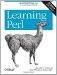# Section 2.1. Numbers

### 2.1. Numbers

Though a scalar is most often either a number or a string, it's useful to look at numbers and strings separately for the moment. We'll cover numbers first and then move on to strings.

#### 2.1.1. All Numbers Have the Same Format Internally

As you'll see in the next few paragraphs, you can specify integers (whole numbers, like 255 or 2001) and floating-point numbers (real numbers with decimal points, like 3.14159, or 1.35x1025). But internally, Perl computes with double-precision floating-point values.[*] This means that there are no integer values internal to Perl. An integer constant in the program is treated as the equivalent floating-point value.[] You probably wont notice the conversion (or care much), but you should stop looking for distinct integer operations (as opposed to floating-point operations) because they don't exist.[]

[] Well, Perl will sometimes use internal integers in ways invisible to the programmer. That is, the only difference you should generally be able to see is that your program runs faster. And who could complain about that?

] Okay, there is the integer pragma. But using that is beyond the scope of this book. And yes, some operations compute an integer from a given floating-point number, as youll see later. But that's not what we're talking about here.

#### 2.1.2. Floating-Point Literals

A literal is the way a value is represented in the source code of the Perl program. A literal is not the result of a calculation or an I/O operation; it's data written directly into the source code.

Perl's floating-point literals should look familiar to you. Numbers with and without decimal points are allowed (including an optional plus or minus prefix), as well as attaching a power-of-10 indicator (exponential notation) with E notation.

`     1.25     255.000     255.0     7.25e45  # 7.25 times 10 to the 45th power (a big number)     -6.5e24  # negative 6.5 times 10 to the 24th              # (a big negative number)     -12e-24  # negative 12 times 10 to the -24th              # (a very small negative number)     -1.2E-23 # another way to say that - the E may be uppercase `

#### 2.1.3. Integer Literals

Integer literals are straightforward:

`     0     2001     -40     255     61298040283768 `

That last one is a little hard to read. Perl allows underscores for clarity within integer literals, so you can also write that number like this:

`     61_298_040_283_768 `

It's the same value but looks different to us human beings. You might have thought that commas should be used for this purpose, but commas are used for a more important purpose in Perl (as you'll see in the next chapter).

#### 2.1.4. Non-Decimal Integer Literals

Like many other programming languages, Perl allows you to specify numbers in bases other than 10 (decimal). Octal (base 8) literals start with a leading 0, hexadecimal (base 16) literals start with a leading 0x, and binary (base 2) literals start with a leading 0b.[*] The hex digits A through F (or a through f) represent the conventional digit values of 10 through 15:

[*] The "leading zero" indicator works only for literals and not for automatic string-to-number conversion, which you'll see later in this chapter. You can convert a data string that looks like an octal or hex value into a number with oct( ) or hex( ). Though there's no "bin" function for converting binary values, oct( ) can do that for strings beginning with 0b.

`     0377       # 377 octal, same as 255 decimal     0xff       # FF hex, also 255 decimal     0b11111111 # also 255 decimal `

Though these values look different to us humans, all three are the same number to Perl. It makes no difference to Perl whether you write 0xFF or 255.000, so choose the representation that makes the most sense to you and your maintenance programmer (by which we mean the poor chap who gets stuck trying to figure out what you meant when you wrote your code. Most often, this poor chap is you, and you can't recall why you did what you did three months ago).

When a non-decimal literal is more than about four characters long, it may be hard to read. For this reason, Perl allows underscores for clarity within these literals:

`     0x1377_0B77     0x50_65_72_7C `

#### 2.1.5. Numeric Operators

Perl provides the typical ordinary addition, subtraction, multiplication, and division operators, and so on:

`     2 + 3      # 2 plus 3, or 5     5.1 - 2.4  # 5.1 minus 2.4, or 2.7     3 * 12     # 3 times 12 = 36     14 / 2     # 14 divided by 2, or 7     10.2 / 0.3 # 10.2 divided by 0.3, or 34     10 / 3     # always floating-point divide, so 3.3333333... `

Perl also supports a modulus operator (%). The value of the expression 10 % 3 is the remainder when 10 is divided by 3, which is 1. Both values are first reduced to their integer values, so 10.5 % 3.2 is computed as 10 % 3.[*] Additionally, Perl provides the FORTRAN-like exponentiation operator, which many have yearned for in Pascal and C. The operator is represented by the double asterisk, such as 2**3, which is two to the third power, or eight.[] We will introduce other numeric operators as we need them.

[] You cant normally raise a negative number to a noninteger exponent. Math geeks know that the result would be a complex number. To make that possible, you'll need the help of the Math::Complex module.Learning Perl, 5th Edition
ISBN: 0596520107
EAN: 2147483647
Year: 2003
Pages: 232

Similar book on Amazon